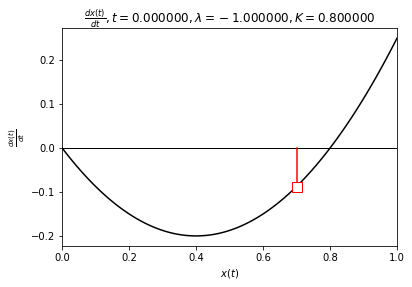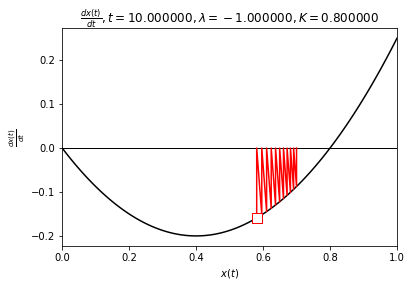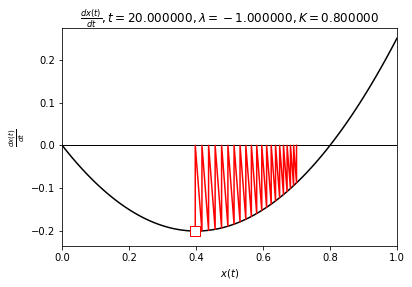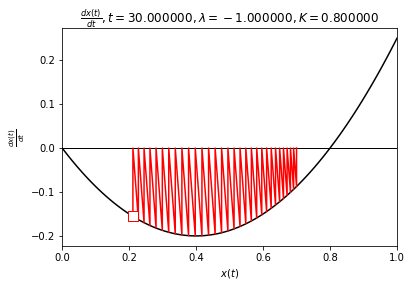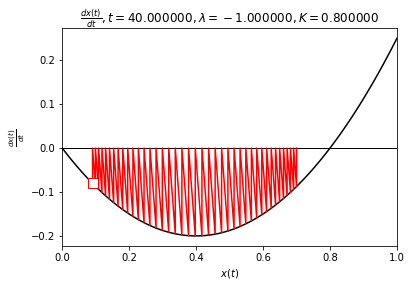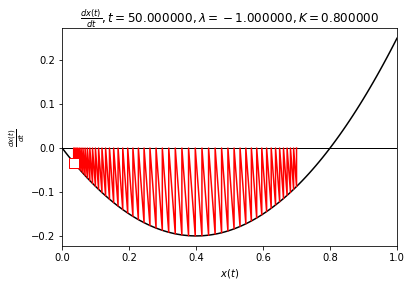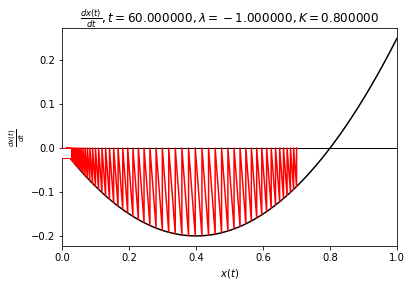# Evolutionary Dynamics - Exercise 1¶

by Daniel Dugas, Florian Tschopp, and Michel Breyer

In :
import matplotlib.pyplot as plt
import numpy as np
import sympy
from sympy.solvers import solve
from sympy import Symbol, Function
from IPython.display import display


## Problem 1: Logistic difference equation¶

In a discrete time model for population growth, the value $x$ (number of cells divided by the maximum number supported by the habitat) at time $t + 1$ is calculated from the value at time $t$ according to the difference equation $$x_{t+1} = r x_t(1−x_t).$$

We will use sympy to derive symbolic solutions. First, we define the update function:

In :
xt = Symbol('x_t')
r = Symbol('r')
def f_(r, xt):
return r * xt * (1 - xt)
f = f_(r, xt)
display(f)

$\displaystyle r x_{t} \left(1 - x_{t}\right)$

Thus the derivative of the update function is:

In :
fprime = sympy.simplify(sympy.diff(f, xt))
display(fprime)

$\displaystyle r \left(1 - 2 x_{t}\right)$

### (a) Determine the equilibrium points $x^*$ of the system¶

We find the equilibrium points by solving $f(x)=x$ for $x$.

In :
equilibrium_points =  sympy.solve(f - xt, xt)

print("Equilibrium points x*:")
for x_star in equilibrium_points:
display(x_star)

Equilibrium points x*:

$\displaystyle 0$
$\displaystyle \frac{r - 1}{r}$

Note that the solution $x^* = (r - 1)/r$ only exists if $r \neq 0$.

### (b) Are the points stable for $r = 0.5$, $r = 1.5$, $r = 2.5$?¶

The condition for stability is $|f'(x^*)|<1$. In our case,

In :
r_values = [0.5, 1.5, 2.5]
for ri in r_values:
print("r = {},".format(ri))
for xi in equilibrium_points:
xi = float(xi.subs(r, ri))
fpi = fprime.subs(xt, xi).subs(r, ri)
is_stable = abs(fpi) < 1
print("  x* = {:>5.2f} : f' = {:>5.2f} -> equilibrium point is {}stable".format(xi, fpi, '' if is_stable else 'un'))

r = 0.5,
x* =  0.00 : f' =  0.50 -> equilibrium point is stable
x* = -1.00 : f' =  1.50 -> equilibrium point is unstable
r = 1.5,
x* =  0.00 : f' =  1.50 -> equilibrium point is unstable
x* =  0.33 : f' =  0.50 -> equilibrium point is stable
r = 2.5,
x* =  0.00 : f' =  2.50 -> equilibrium point is unstable
x* =  0.60 : f' = -0.50 -> equilibrium point is stable


### (c) Confirm this by numerically iterating the difference equation.¶

For each value of $r$, we iterate the difference equation for $100$ steps with initial conditions close to the two fixed points.

In :
r_values = [0.5, 1.5, 2.5]
for ri in r_values:
print("r = {},".format(ri))
for xi in equilibrium_points:
xi = float(xi.subs(r, ri))
fpi = fprime.subs(xt, xi).subs(r, ri)
is_stable = fpi > 0
print("  x* = {:>5.2f} :".format(xi))
t = 0
eps = 0.01
x_t = xi + eps
print("    Initial x = {}, (epsilon = {})".format(x_t, eps))
for t in range(100):
x_t = float(f.subs(r, ri).subs(xt, x_t))
is_close = ( x_t - xi ) < eps
is_close_string = "" if is_close else " -> has shifted from starting equilibrium!"
print("    After {} iterations, x = {:.2f}{}".format(t+1, x_t, is_close_string))

r = 0.5,
x* =  0.00 :
Initial x = 0.01, (epsilon = 0.01)
After 100 iterations, x = 0.00
x* = -1.00 :
Initial x = -0.99, (epsilon = 0.01)
After 100 iterations, x = -0.00 -> has shifted from starting equilibrium!
r = 1.5,
x* =  0.00 :
Initial x = 0.01, (epsilon = 0.01)
After 100 iterations, x = 0.33 -> has shifted from starting equilibrium!
x* =  0.33 :
Initial x = 0.3433333333333333, (epsilon = 0.01)
After 100 iterations, x = 0.33
r = 2.5,
x* =  0.00 :
Initial x = 0.01, (epsilon = 0.01)
After 100 iterations, x = 0.60 -> has shifted from starting equilibrium!
x* =  0.60 :
Initial x = 0.6100000000000001, (epsilon = 0.01)
After 100 iterations, x = 0.60


We observe that the sequences diverge from the repelling fixed points.

### (d) Examine the stability and behaviour for $r = 3.5$.¶

Hint: Plot the Poincaré section of $x_t$ against $x_{t−1}$, and remove transient points.

First, we examine the derivative at the equilibrium points.

In :
r_values = [3.5]
for ri in r_values:
print("r = {},".format(ri))
for xi in equilibrium_points:
xi = float(xi.subs(r, ri))
fpi = fprime.subs(xt, xi).subs(r, ri)
is_stable = abs(fpi) < 1
print("  x* = {:>5.2f} : f' = {:>5.2f} -> equilibrium point is {}stable".format(xi, fpi, '' if is_stable else 'un'))

r = 3.5,
x* =  0.00 : f' =  3.50 -> equilibrium point is unstable
x* =  0.71 : f' = -1.50 -> equilibrium point is unstable


We see that for $r = 3.5$, both equilibrium points are unstable.

Tracing two sequences on a Poincaré plot also shows that the paths diverge from the fixed points.

In :
N = 10
xs1, xs2 = np.zeros(N), np.zeros(N)

xs1 = 0.01
xs2 = 0.70

for t in range(N-1):
xs1[t+1] = f_(3.5, xs1[t])
xs2[t+1] = f_(3.5, xs2[t])

fig, (ax1, ax2) = plt.subplots(1, 2)

ax1.plot(xs1[:-1], xs1[1:], 'o-', label='$x_0$=0.01')
ax1.plot([0, 1], [0, 1])
ax1.set_xlabel('$x_{t-1}$')
ax1.set_ylabel('$x_{t}$')
ax1.axis('equal')
ax1.legend()

ax2.plot(xs2[:-1], xs2[1:], 'o-', label='$x_0$=0.70')
ax2.plot([0, 1], [0, 1])
ax2.set_xlabel('$x_{t-1}$')
ax2.axis('equal')
ax2.legend()

plt.show()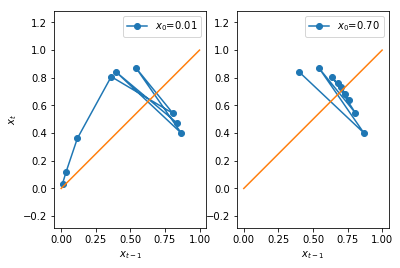### (e) What happens for $r = 3.9$?¶

Again, we compute the derivative at $x^*$ for $r=3.9$.

In :
ri = 3.9
print("r = {},".format(ri))

for xi in equilibrium_points:
xi = float(xi.subs(r, ri))
fpi = float(fprime.subs(xt, xi).subs(r, ri))
is_stable = abs(fpi) < 1
print("  x* = {:>5.2f} : f' = {:>5.2f} -> equilibrium point is {}stable".format(xi, fpi, '' if is_stable else 'un'))

r = 3.9,
x* =  0.00 : f' =  3.90 -> equilibrium point is unstable
x* =  0.74 : f' = -1.90 -> equilibrium point is unstable


For visualization, we plot 10 iterations of the update function with a random initial condition.

In :
x = np.linspace(0.0, 1.0, 100)
fx = f_(ri, x)

# Iterate
N = 10
xs = np.zeros((N,))
xs = 0.02  # np.random.rand()
for t in range(N-1):
xs[t+1] = f_(ri, xs[t])

# Generate plots
plot_x = []
plot_y = []

Y = xs
for t in range(N-1):
X = xs[t]
plot_x.append(X)
plot_y.append(Y)
Y = xs[t+1]
plot_x.append(X)
plot_y.append(Y)

plt.figure()
plt.plot(x, fx, color='k')
plt.plot([0, 1],[0, 1], color='k', linewidth=1)
plt.plot(plot_x, plot_y, color='red')
plt.scatter(X, Y, marker="s", s=100, edgecolor="red", facecolor="white", zorder=4)
plt.text(0.85, 0.8, "$x = f(x)$")

plt.xlim(0.0, 1.0)
plt.ylim(0.0, 1.0)
plt.title("Iteration {}".format(t))
plt.xlabel("$x$")
plt.ylabel("$f(x)$")

plot_x.append(Y)
plot_y.append(Y)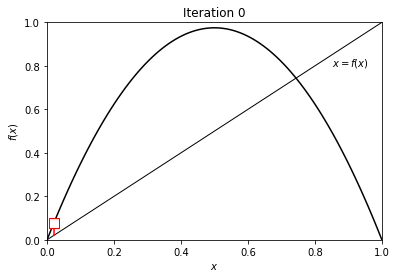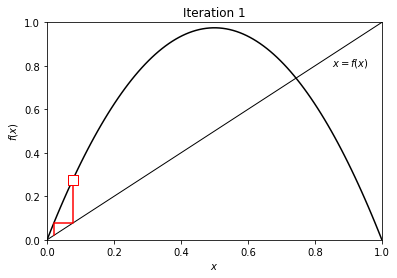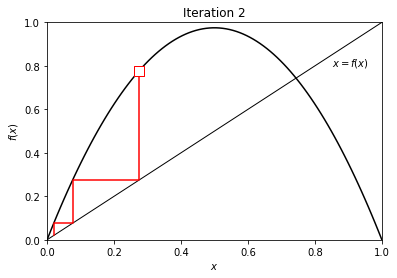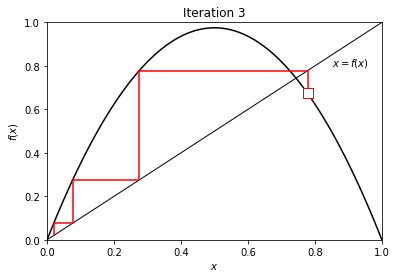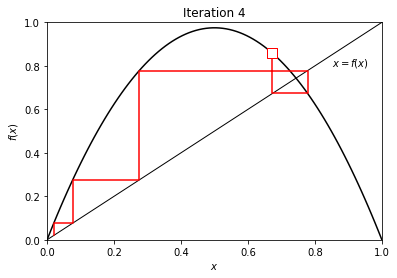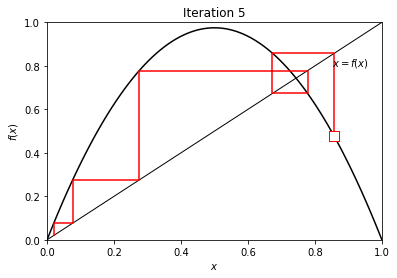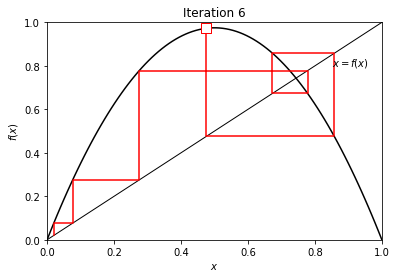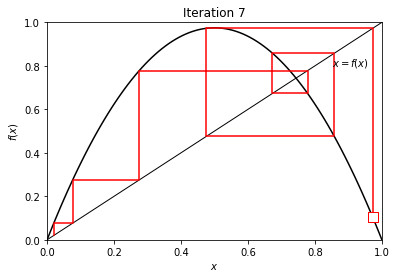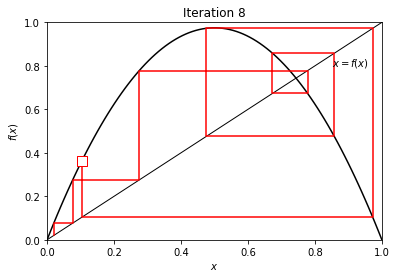At $r=3.9$, neither equilibrium points are stable (repellors). This results in oscillating behavior, as visible above.

Note: above, we discuss the difference between equilibrium points with a derivative |f'| > 1 and |f'| < 1.

While the |f'| < or > 1 determines wether they are stable or not, it is interesting to ponder what the effect of f' < 0 and > 0 is on the evolution around the equilibrium.

In :
for ri in [1.5, 2.5]:
print("r = {},".format(ri))
for xi in equilibrium_points:
xi = float(xi.subs(r, ri))
fpi = float(fprime.subs(xt, xi).subs(r, ri))
is_stable = abs(fpi) < 1
print("  x* = {:>5.2f} : f' = {:>5.2f} -> equilibrium point is {}stable".format(xi, fpi, '' if is_stable else 'un'))
focus_equ = xi
focus_equ_x_tp1 = f_(ri, focus_equ)

x_in = np.linspace(-0.1, 1.1, 100)
x_out = f_(ri, x_in)
# iterate starting from a random point
x_0 = focus_equ - 0.05 # np.random.rand()
t = 0
x_t = x_0
N = 10
xx = np.zeros((N,))
f_ri = f.subs(r, ri)
for t in range(N):
xx[t] = x_t
x_t = float(f_ri.subs(xt, x_t))
# make a stepping plot of the iterations
Y = x_0
plot_x = []
plot_y = []
for t in range(N-1):
X = xx[t]
plot_x.append(X)
plot_y.append(Y)
Y = xx[t+1]
plot_x.append(X)
plot_y.append(Y)
# new figure
plt.figure()
plt.plot(x_in, x_out, color='k')
plt.axhline(linewidth=1, color='k')
plt.axhline(focus_equ_x_tp1, linewidth=1, color='k')
plt.axvline(focus_equ, linewidth=1, color='k')
plt.plot([-1, 1],[-1, 1], color='k', linewidth=1)
plt.xlim([focus_equ-0.1, focus_equ+0.1])
plt.ylim([focus_equ_x_tp1-0.1, focus_equ_x_tp1+0.1])
plt.title("Around equilibrium point x* = {:.2f}, f' = {:.2f}".format(focus_equ, fpi))
plt.xlabel("$x$")
plt.ylabel("$f(x)$")
plt.plot(plot_x, plot_y, color='red')
plt.scatter(X, Y, marker="s", s=100, edgecolor="red", facecolor="white", zorder=4)
plot_x.append(Y)
plot_y.append(Y)

r = 1.5,
x* =  0.00 : f' =  1.50 -> equilibrium point is unstable
x* =  0.33 : f' =  0.50 -> equilibrium point is stable
r = 2.5,
x* =  0.00 : f' =  2.50 -> equilibrium point is unstable
x* =  0.60 : f' = -0.50 -> equilibrium point is stable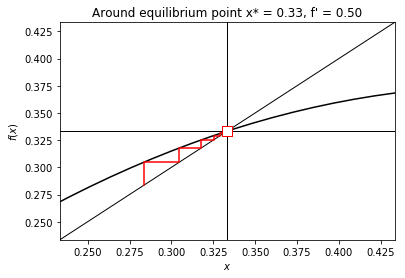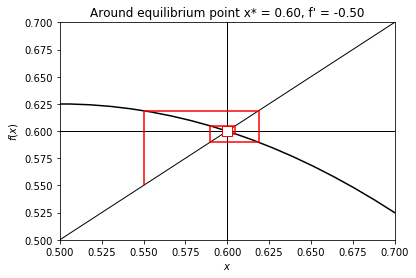Above visible, is the difference between two stable equilibrium points, one where 0 < f' < 1 and one where -1 < f' < 0.

We note that though both are stable, around the stable equilibrium point with f' < 0 we see oscillating ('overshooting') behavior which nonetheless converges, while around the stable equilibrium point with f' > 0, x_t approaches the equilibrium monotonically from one side.

## Problem 2: Logistic growth in continuous time¶

The logistic model for population growth is:

In :
Lambda = Symbol('lamda')
T = Symbol('t')
X_of_t = Function('x')(T)
K = Symbol('K')
X_0 = Symbol('x_0')
Exp = sympy.exp

In :
Dx_dt = Lambda * X_of_t * ( 1 - (X_of_t / K ) )
Df_dx = sympy.diff(Dx_dt, X_of_t)

In :
Dx_dt

Out:
$\displaystyle \lambda \left(1 - \frac{x{\left(t \right)}}{K}\right) x{\left(t \right)}$

### (a) Show, by direct integration of (1), that the solution is given by:¶

Hint: Use separation of variables and partial fractions.

Separation of variables: $$\int{\lambda dt} = \int{\frac{dx(t)}{x(t)(1 - \frac{x(t)}{K})}}$$

Partial fractions (rhs): $$\int{\frac{K}{x(t) (K - x(t))}dx(t)} = \int{\frac{K - x(t)}{x(t) (K - x(t))} + \frac{x(t)}{x(t) (K - x(t))}dx(t)} = \int{\frac{1}{x(t)}+\frac{1}{K - x(t)}dx(t)}$$

As a result: $$\int{\lambda dt} = \int{\frac{1}{x(t)}dx(t)} + \int{\frac{1}{K - x(t)}dx(t)}$$

Of which all integrals have known solutions: $$\int{\lambda dt} \rightarrow kt + constant$$ $$\int{\frac{1}{x(t)}dx(t)} \rightarrow ln \left | x(t) \right | + constant$$ $$\int{\lambda dt} \rightarrow -ln \left | K - x(t) \right | + constant$$

Thus, $$\lambda t + C = ln \left | x(t) \right | - ln \left | K - x(t) \right | = ln \left | \frac{x(t)}{K - x(t)} \right |$$

Exponential on both sides: $$e^{\lambda t} e^{C} = \left | \frac{x(t)}{K - x(t)} \right |$$

To find exp(C) we set t to 0: $$e^{C} = \left | \frac{x_0}{K - x_0} \right |$$

Thus: $$e^{\lambda t} \left | \frac{x_0}{K - x_0} \right | =\left | \frac{x(t)}{K - x(t)} \right |$$

Multiplying both sides by the r.h.s denominator, and developping: $$(K - x(t)) e^{\lambda t} \frac{\pm x_0}{K - x_0} = x(t),$$

$$K e^{\lambda t} \frac{\pm x_0}{K - x_0} = x(t) + e^{\lambda t} \frac{\pm x_0}{K - x_0} x(t)$$

Which gives: $$x(t) = \frac{K e^{\lambda t} \frac{\pm x_0}{K - x_0}}{1 +e^{\lambda t} \frac{\pm x_0}{K - x_0}}$$

We now multiply the r.h.s denominator and numerator by K - x_0: $$x(t) = \frac{K x_0 e^{\lambda t}}{K - x_0 +e^{\lambda t} x_0}$$

Finally, factorizing x_0 in the r.h.s denominator, we obtain $$x(t) = \frac{K x_0 e^{\lambda t}}{K + x_0 ( e^{\lambda t} - 1) }$$

### (b) Find the equilibrium points of the system and discuss their stability.¶

The condition for equilibrium is $\frac{dx(t)}{dt} = 0$. Solving the equation yields:

In :
equilibrium_points = sympy.solve(Dx_dt, X_of_t)
print("Equilibrium points are:")
for xi in equilibrium_points:
print("  x* = {}".format(xi))

Equilibrium points are:
x* = 0
x* = K


The condition for a stable equilibrium is $\frac{df}{dx}|_{x=x^*} < 0$.

Let's evaluate $\frac{df}{dx}$ at both equilibrium points.

In :
for xi in equilibrium_points:
print("Equilibrium point x* = {}, df/dx|x=x*:".format(xi))
display(Df_dx.subs(X_of_t, xi))

Equilibrium point x* = 0, df/dx|x=x*:

$\displaystyle \lambda$
Equilibrium point x* = K, df/dx|x=x*:

$\displaystyle - \lambda$
In :
yy = []
xx = np.linspace(-0.1, 1.1, 100)
ll = [1, -1]
ki = 0.8
for li in ll:
yy = []
for xi in xx:
yy.append(Dx_dt.subs(X_of_t, xi).subs(Lambda, li).subs(K, ki))
yy = np.array(yy)
plt.figure()
plt.plot(xx, yy, color='k')
plt.axhline(linewidth=1, color='k')
for x_star in equilibrium_points:
plt.axvline(x_star.subs(K, ki), linewidth=1, color='r')
plt.xlim([-0.1, 1.2])
plt.title(r"$\frac{dx(t)}{dt}, \lambda = %f, K = %f$" % (li, ki))
plt.xlabel(r"$x(t)$")
plt.ylabel(r"$\frac{dx(t)}{dt}$")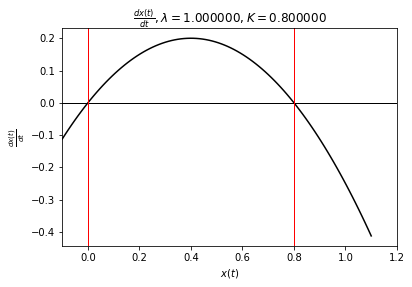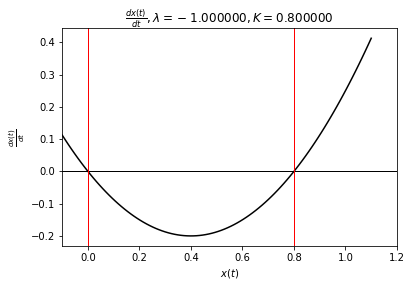We conclude that there exist one stable and one unstable equilibrium, which-is-which depending on whether $\lambda$ is positive or negative.

• For positive lambda, $x^* = 0$ is unstable, while $x^* = K$ is stable.
• For negative lambda, $x^* = 0$ is stable, while $x^* = K$ is unstable.

This makes intuitive sense as for an x slightly away from the equilibrium (let us call epsilon the distance between x and x*), a positive slope in d(dx(t)/dt)/dx(t) means that the change in x is of the same sign as epsilon. Thus, the absolute value of epsilon will increase.

### (c) Numerically integrate to demonstrate the results above.¶

We will integrate the equation for $K=1$ using RK45.

In :
from scipy.integrate import solve_ivp

def integrate(lam):
# Integrate the ODE for two different initial conditions
f = lambda t, x, : lam * x * (1 - x)
t_span = (0.0, 40.0)

fig, ax = plt.subplots(1)

x0 = [0.2]
sol = solve_ivp(f, t_span, x0)
ax.plot(sol.t, sol.y.squeeze(), label='$x_0$=0.2')

x0 = [0.8]
sol = solve_ivp(f, t_span, x0)
ax.plot(sol.t, sol.y.squeeze(), label='$x_0$=0.8')

ax.set_xlabel('$t$')
ax.set_ylabel('$x(t)$')
plt.legend()
plt.show()


In case of a positive $\lambda$, the solutions converge to $x^*=K$

In :
integrate(lam=0.5)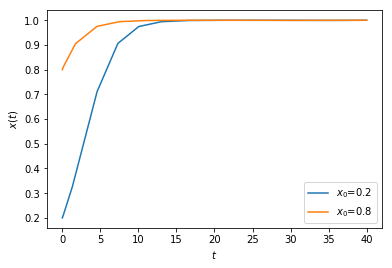In case of a negative $\lambda$, the solutions converge to $x^*=0$.

In :
integrate(lam=-0.5)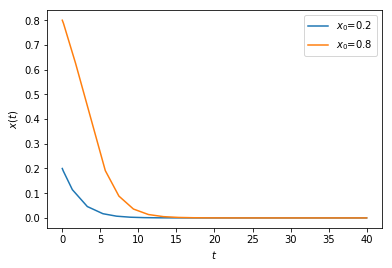Alternatively, we can integrate the equation using 1st order Euler

$$x_{t+1} = x_{t} + \frac{dx(t_t)}{dt} \Delta t$$ $$t_{t+1} = t_t + \Delta t$$

and trace the iterations:

In :
x_axis = np.linspace(0.0, 1.0, 100)
ki = 0.8
li = 1
y_axis = []
for xi in x_axis:
y_axis.append(Dx_dt.subs(X_of_t, xi).subs(Lambda, li).subs(K, ki))
y_axis = np.array(y_axis)
# iterate starting from a random point
x_0 = 0.1 # np.random.rand()
t = 0
dt = 0.1
x_t = x_0
N = 70
plot_every = 10
xx = np.zeros((N,))
dxx = np.zeros((N,))
for t in range(N):
xx[t] = x_t
dxx[t] = float(Dx_dt.subs(K, ki).subs(Lambda, li).subs(X_of_t, x_t))
x_t = x_t + dxx[t] * dt

print("We now numerically integrate with K = {}, Lambda = {}, dt = {}".format(ki, li, dt))
# make a stepping plot of the iterations
Y = x_0
plot_x = []
plot_y = []
for t in range(N-1):
X = xx[t]
Y = dxx[t]
plot_x.append(X)
plot_y.append(0)
plot_x.append(X)
plot_y.append(Y)
yy = []
if t % plot_every == 0:
plt.figure()
plt.plot(x_axis, y_axis, color='k')
plt.axhline(linewidth=1, color='k')
plt.xlim(0.0, 1.0)
plt.title(r"$\frac{dx(t)}{dt}, t = %f, \lambda = %f, K = %f$" % (t, li, ki))
plt.xlabel(r"$x(t)$")
plt.ylabel(r"$\frac{dx(t)}{dt}$")
plt.plot(plot_x, plot_y, color='red')
plt.scatter(X, Y, marker="s", s=100, edgecolor="red", facecolor="white", zorder=4)
plot_x.append(X + Y * dt)
plot_y.append(0)

We now numerically integrate with K = 0.8, Lambda = 1, dt = 0.1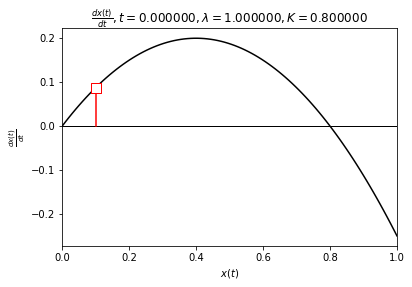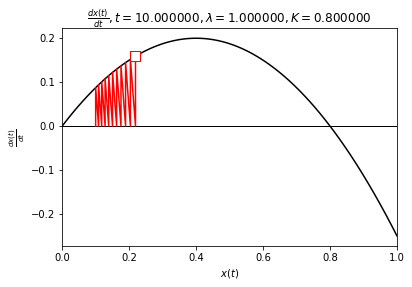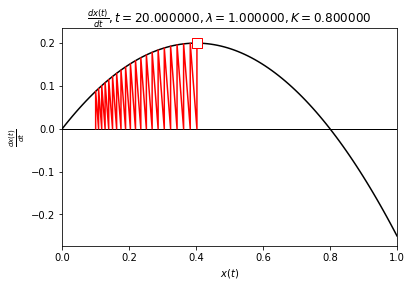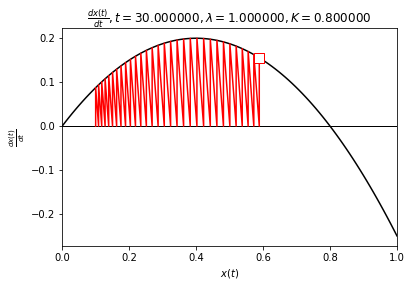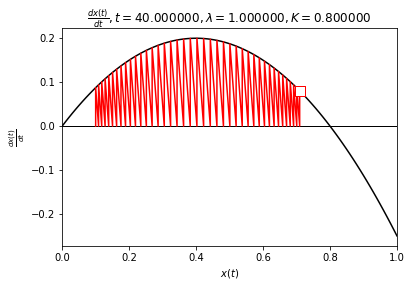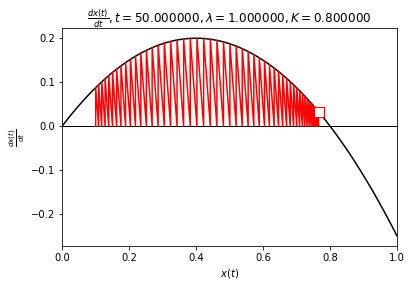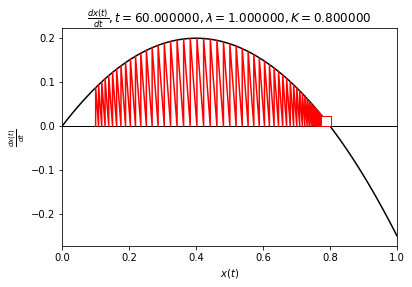As visible above, though x_0 is closer to the equilibrium point a x* = 0, after numerical integration the population ends up converging to x* = K, the only stable equilibrium point (assuming lambda > 0). This result confirms the results in question (b)

Below is the same operation for the case where lambda is negative. We observe that the stability of the equilibrium points are now reversed.

In :
x_axis = np.linspace(0.0, 1.0, 100)
ki = 0.8
li = -1
y_axis = []
for xi in x_axis:
y_axis.append(Dx_dt.subs(X_of_t, xi).subs(Lambda, li).subs(K, ki))
y_axis = np.array(y_axis)
# iterate starting from a random point
x_0 = 0.7 # np.random.rand()
t = 0
dt = 0.1
x_t = x_0
N = 70
plot_every = 10
xx = np.zeros((N,))
dxx = np.zeros((N,))
for t in range(N):
xx[t] = x_t
dxx[t] = float(Dx_dt.subs(K, ki).subs(Lambda, li).subs(X_of_t, x_t))
x_t = x_t + dxx[t] * dt

print("We now numerically integrate with K = {}, Lambda = {}, dt = {}".format(ki, li, dt))
# make a stepping plot of the iterations
Y = x_0
plot_x = []
plot_y = []
for t in range(N-1):
X = xx[t]
Y = dxx[t]
plot_x.append(X)
plot_y.append(0)
plot_x.append(X)
plot_y.append(Y)
yy = []
if t % plot_every == 0:
plt.figure()
plt.plot(x_axis, y_axis, color='k')
plt.axhline(linewidth=1, color='k')
plt.xlim(0.0, 1.0)
plt.title(r"$\frac{dx(t)}{dt}, t = %f, \lambda = %f, K = %f$" % (t, li, ki))
plt.xlabel(r"$x(t)$")
plt.ylabel(r"$\frac{dx(t)}{dt}$")
plt.plot(plot_x, plot_y, color='red')
plt.scatter(X, Y, marker="s", s=100, edgecolor="red", facecolor="white", zorder=4)
plot_x.append(X + Y * dt)
plot_y.append(0)

We now numerically integrate with K = 0.8, Lambda = -1, dt = 0.1